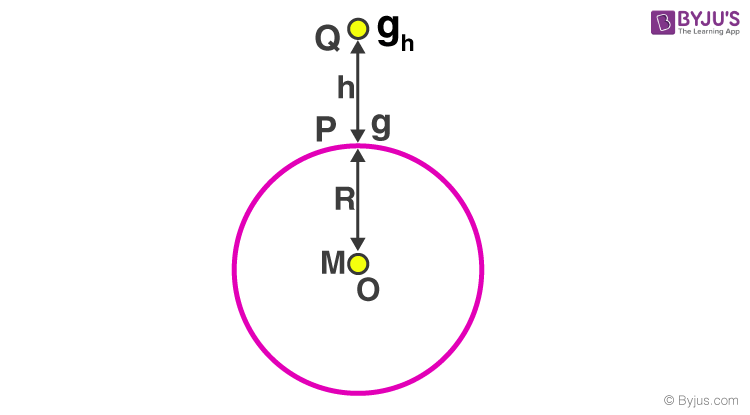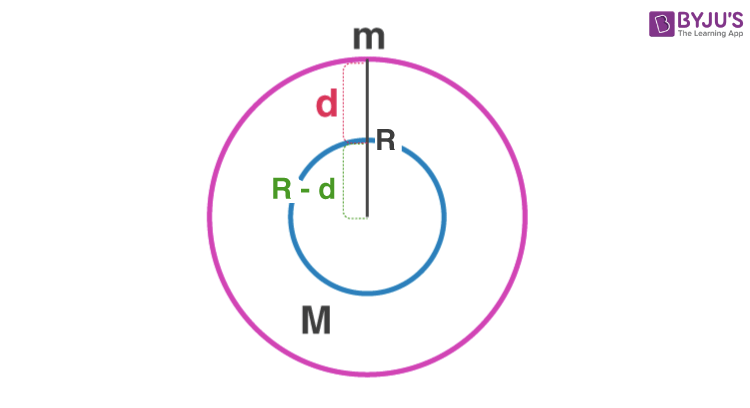Win up to 100% scholarship on Aakash BYJU'S JEE/NEET courses with ABNAT Win up to 100% scholarship on Aakash BYJU'S JEE/NEET courses with ABNAT

# Acceleration due to Gravity - Value of g on Earth

## What Is Acceleration Due to Gravity?

Acceleration due to gravity is the acceleration gained by an object due to gravitational force. Its SI unit is m/s2. It has both magnitude and direction; hence, it’s a vector quantity. Acceleration due to gravity is represented by g. The standard value of g on the surface of the earth at sea level is 9.8 m/s2.

### JEE Main 2021 LIVE Physics Paper Solutions 24 Feb Shift-1 Memory-based### Acceleration Due to Gravity – Formula, Unit and Values

 Acceleration Due to Gravity (g) Symbol g Dimensional Formula M0L1T-2 SI Unit ms-2 Formula g = GM/r2 Values of g in SI 9.806 ms-2 Values of g in CGS 980 cm s-2

## What Is Gravity?

Gravity is the force with which the earth attracts a body towards its centre. Let us consider two bodies of masses, ma and mb. Under the application of equal forces on two bodies, the force in terms of mass is given by

mb = m[aA/aB]; this is called an inertial mass of a body.

Under the gravitational influence on two bodies,

• FA = GMmA/r2,
• FB = GMmB/r2,
• mB = [FB/FA] × mA

⇒ More on Gravitation:

The above mass is called a gravitational mass of a body. According to the principle of equivalence, the inertial mass and gravitational mass are identical. We will be using this while deriving acceleration due to the gravity given below.

Suppose a body [test mass (m)] is dropped from a height ‘h’ above the surface of the earth [source mass (M)]; it begins to move downwards with an increase in velocity as it reaches close to the earth’s surface.

We know that the velocity of an object changes only under the action of a force; in this case, the force is provided by gravity.

Under the action of gravitational force, the body begins to accelerate toward the earth’s centre, which is at a distance ‘r’ from the test mass.

Then, ma = GMm/r2 (Applying principle of equivalence)

⇒ a = GM/r2 . . . . . . . (1)

The above acceleration is due to the gravitational pull of the earth, so we call it acceleration due to gravity; it does not depend upon the test mass. Its value near the surface of the earth is 9.8 ms-2.

Therefore, the acceleration due to gravity (g) is given by = GM/r2.

## Formula of Acceleration Due to Gravity

Force acting on a body due to gravity is given by f = mg

Where f is the force acting on the body, g is the acceleration due to gravity, and m is the mass of the body.

According to the universal law of gravitation, f = GmM/(r+h)2

Where,

• f = Force between two bodies
• G = Universal gravitational constant (6.67×10-11 Nm2/kg2)
• m = Mass of the object
• M = Mass of the earth
• r = Radius of the earth
• h = Height at which the body is from the surface of the earth

As the height (h) is negligibly small compared to the radius of the earth, we re-frame the equation as follows:

f = GmM/r2

Now, equating both expressions,

mg = GmM/r2

⇒ g = GM/r2

Therefore, the formula of acceleration due to gravity is given by g = GM/r2

Note: It depends on the mass and radius of the earth.

This helps us understand the following:

• All bodies experience the same acceleration due to gravity, irrespective of their mass.
• Its value on earth depends upon the mass of the earth and not the mass of the object.

## Acceleration Due to Gravity on the Surface of Earth

Earth is assumed to be a uniform solid sphere with a mean density. We know that,

Density = mass/volume

Then, ρ = M/[4/3 πR3]

⇒ M = ρ × [4/3 πR3]

We know that g = GM/R2.

On substituting the values of M, we get,

g = 4/3 [πρRG]

At any distance ‘r’ from the centre of the earth,

g = 4/3 [πρRG]

The value of  acceleration due to gravity ‘g’ is affected by

• Altitude above the earth’s surface.
• Depth below the earth’s surface.
• The shape of the earth.
• Rotational motion of the earth.

## Variation of g with HeightAcceleration due to gravity at a height (h) from the surface of the earth

Consider a test mass (m) at a height (h) from the surface of the earth. Now, the force acting on the test mass due to gravity is

F = GMm/(R+h)2

Where M is the mass of the earth, and R is the radius of the earth. The acceleration due to gravity at a certain height is ‘h’, then

mgh= GMm/(R+h)2

⇒ gh= GM/[R2(1+ h/R)2 ] . . . . . . (2)

The acceleration due to gravity on the surface of the earth is given by

g = GM/R2 . . . . . . . . . (3)

On dividing equations (3) and (2), we get

g= g (1+h/R)-2. . . . . . (4)

This is the acceleration due to gravity at a height above the surface of the earth. Observing the above formula, we can say that the value of g decreases with an increase in the height of an object, and the value of g becomes zero at an infinite distance from the earth.

Approximation Formula:

From equation (4)

when h << R, the value of g at height ‘h’ is given by g= g/(1 – 2h/R)

## Variation of g with DepthConsider a test mass (m) taken to a distance (d) below the earth’s surface, the acceleration due to gravity at that point (gd) is obtained by taking the value of g in terms of density.

On the surface of the earth, the value of g is given by

g = 4/3 × πρRG

At a distance (d) below the earth’s surface, the acceleration due to gravity is given by

gd = 4/3 × πρ × (R – d) G

On dividing the above equations, we get,

gd = g (R – d)/R

• When the depth d = 0, the value of g on the surface of the earth gd = g.
• When the depth d = R, the value of g at the centre of the earth gd = 0.

### Variation of g Due to the Shape of the Earth

As the earth is an oblate spheroid, its radius near the equator is more than its radius near the poles. Since for a source mass, the acceleration due to gravity is inversely proportional to the square of the radius of the earth, it varies with latitude due to the shape of the earth.

gp/ge = R2e/R2p

Where ge and gp are the accelerations due to gravity at the equator and poles, Rand Rp are the radii of the earth near the equator and poles.

From the above equation, it is clear that acceleration due to gravity is more at the poles and less at the equator. So if a person moves from the equator to the poles, their weight decreases as the value of g decreases.

### Variation of g Due to Rotation of Earth

Consider a test mass (m) is on a latitude making an angle with the equator. As we have studied, when a body is under rotation, every particle in the body makes circular motions about the axis of rotation. In the present case, the earth is under rotation with a constant angular velocity ω, then the test mass moves in a circular path of radius ‘r’ with an angular velocity ω.

This is the case of a non-inertial frame of reference, so there exists a centrifugal force on the test mass (mrω2). Gravity is acting on the test mass towards the centre of the earth (mg).

As both these forces are acting from the same point, these are known as co-initial forces, and as they lie along the same plane, they are termed co-planar forces.

We know from the parallelogram law of vectors if two co-planar vectors are forming two sides of a parallelogram, then the resultant of those two vectors will always be along the diagonal of the parallelogram.

Applying the parallelogram law of vectors, we get the magnitude of the apparent value of the gravitational force at the latitude.

(mg′)2 = (mg)2 + (mrω2)2 + 2(mg) (mrω2) cos(180 – θ) . . . . . . (1)

We know ‘r’ is the radius of the circular path and ‘R’ is the radius of the earth, then r = Rcosθ.

Substituting r = R cosθ. we get,

g′ = g – Rω2cos2θ

Where g′ is the apparent value of acceleration due to gravity at the latitude due to the rotation of the earth, and g is the true value of gravity at the latitude without considering the rotation of the earth.

At poles, θ = 90°⇒ g’ = g.

At the equator, θ = 0° ⇒ g′= g – Rω2.

### Important Conclusions on Acceleration Due to Gravity

• For an object placed at a height of h, the acceleration due to gravity is less as compared to that placed on the surface.
• As depth increases, the value of acceleration due to gravity (g) falls.
• The value of g is more at the poles and less at the equator.

### Gravitation## Gravitational Potential Energy## Frequently Asked Questions on Acceleration Due to Gravity

Q1

### What does the value 9.8 m/s2 for acceleration due to gravity imply?

The value 9.8 m/s2 for acceleration due to gravity implies that for a freely falling body, the velocity changes by 9.8 m/s every second.

Q2

### Does mass have any effect on acceleration due to gravity?

The acceleration due to gravity is independent of the mass of the body.

Q3

### What is the formula to calculate the force of attraction between two objects?

If m1 and m2 are the two masses separated by a distance r. According to the universal law of gravitation, the force of attraction between them is

F = G (m1 m2/r2)

Q4

### What is free fall?

If the object moves only under the influence of gravity, it is called free fall.

Test your knowledge on Acceleration Due To Gravity